# R语言基础教程5：图形页面排版

### 一、图形边距：mar/mai参数

R绘图参数中表示距离大小的一般都有“英寸”和“行”两种单位方式。图形边距设定参数可以用mai（英寸边距）和mar（行边距）。四个边距的顺序是下、左、上、右，如下图所示：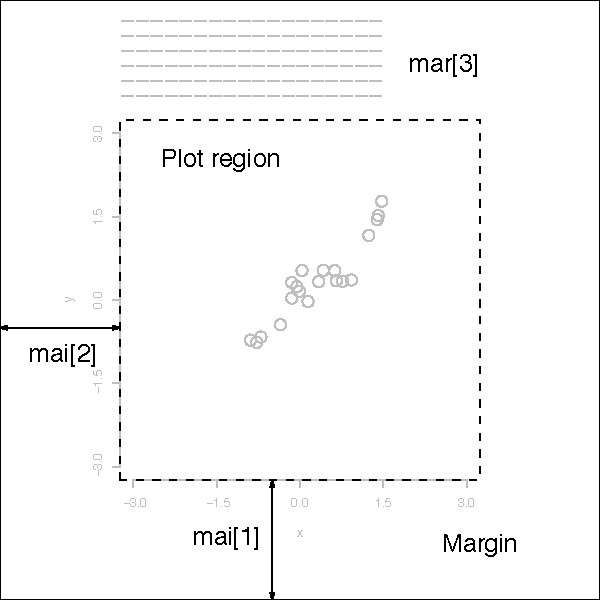```> par(bg="cyan") #把图形背景设为青色以便观察
> par(mar=c(4, 4, 0.5, 0.5)) #设定下边距和左边距为4行，另外两边距为0.5行。
> set.seed(1000) #设此参数是为了下面的rnorm()函数都能获得相同数据
> plot(rnorm(10))```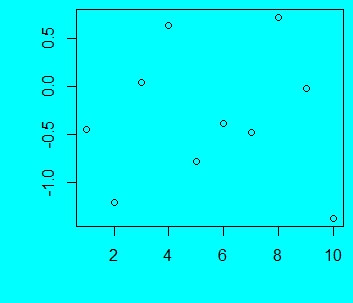mgp参数可设三个数值，依次为标题、刻度标签和刻度的位置。mar/mai设定参数后，图形区（用box函数绘制的边框）边缘位置即为0。所以，mgp=c(2, 0.5, 0)就表示标题在第二行，刻度标签在0.5行，刻度线在0行。

```> par(bg="cyan")
> par(mar=c(4, 4, 0.5, 0.5))
> set.seed(1000)
> par(mgp=c(2,0.5,0)) #坐标轴各项的位置设定
> plot(rnorm(10))```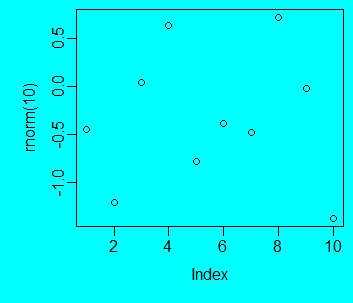### 三、获取位置坐标

```> usr <- par("usr")
> usr
  0.6400000 10.3600000 -1.4568325  0.8034654```

```> xy <- locator(n=1) #参数n表示要获得n个点的坐标，需要点击n次鼠标
> xy
\$x
 -1.430878

\$y
 0.3818771```

### 四、在图形区外添加图例和文字

```> par(mar=c(3, 3, 0.5, 4)) #注意给图例留出空间
> par(mgp=c(2,0.5,0))
> NF <- c(17.44 , 2.56 , 2.70 , 18.71 , 5.61 , 32.98)
> CA <- c(11.48 , 0.75 , 1.16 , 12.73 , 2.84 , 20.04)
> labels <- paste("S", 1:length(NF),sep="")
> the.data <- rbind(NF, CA)
> barplot(the.data, ylim=c(0, max(NF,CA)*1.2), col=c("orange", "red"),
+ xlab="Sample", ylab="Level", beside=TRUE,
+ offset=0, axis.lty=1, names.arg=labels)
> box()```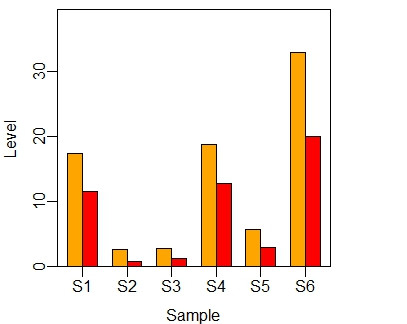```> usr <- par("usr")
> x <- usr*1.02 #图例左上角的x坐标
> y <- usr*0.6 #图例左上角的y坐标
> legend(x, y, legend=c("NF","CA"), fill=c("orange", "red"), box.col="white", xpd=TRUE)```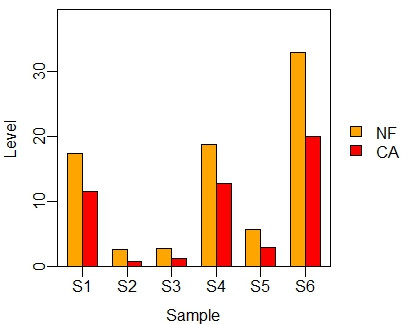`> legend("right", legend=c("NF","CA"), box.col="white", fill=c("orange", "red"), inset=-0.28, xpd=TRUE)`

```> par(mar=c(3, 3, 2, 0.5))
> par(mgp=c(2,0.5,0))
> barplot(the.data, ylim=c(0, max(NF,CA)*1.2), col=c("orange", "red"),
+ xlab="Sample", ylab="Level", beside=TRUE,
+ offset=0, axis.lty=1, names.arg=labels)
> legend("top", legend=c("NF","CA"), box.col="white", fill=c("orange", "red"),
+ ncol=2, inset=-0.15, xpd=TRUE)
> box()```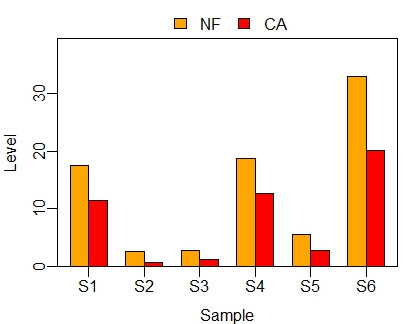```> NF <- c(17.44 , 2.56 , 2.70 , 18.71 , 5.61 , 32.98)
> CA <- c(11.48 , 0.75 , 1.16 , 12.73 , 2.84 , 20.04)
> the.data <- rbind(NF, CA)
> labels <- paste("Sample", 1:length(NF))
> par(mar=c(4, 3, 0.5, 0.5))
> par(mgp=c(2,0.5,0))
> position <- barplot(the.data, ylim=c(0, max(NF,CA)*1.2),
+ col=c("orange", "red"), legend.text=c("NF","CA"),
+ args.legend=list(x="topleft", box.col="white", inset=0.02),
+ ylab="Level", beside=TRUE, offset=0, axis.lty=1)
> #以下为横坐标标签设定
> x <- (position[1,] + position[2,])/2
> y <- par("usr")-1
> text(x, y, labels=labels, adj=1, srt=45, xpd=TRUE)
> box()```

###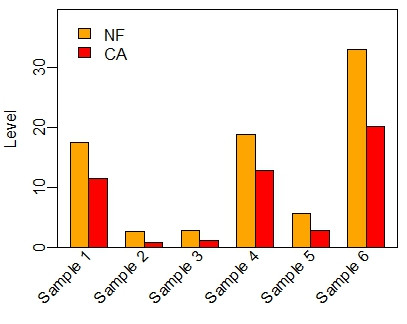五、一页多图

```par(mfrow=c(2,2))
par(mar=c(3, 3, 0.5, 0.5))
set.seed(1000)
plot(rnorm(100),pch="1", cex=0.6)
set.seed(1001)
plot(rnorm(100),pch="2", cex=0.6)
set.seed(100)
plot(rnorm(100),pch="3", cex=0.6)
set.seed(1003)
plot(rnorm(100),pch="4", cex=0.6)```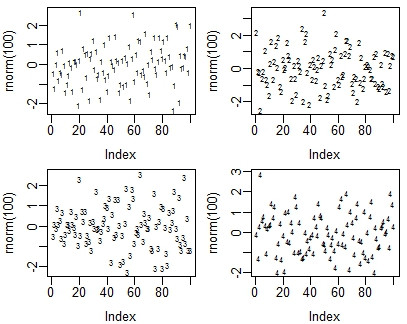```par(mfcol=c(2,2))
par(mar=c(3, 3, 0.5, 0.5))
set.seed(1000)
plot(rnorm(100),pch="1", cex=0.6)
set.seed(1001)
plot(rnorm(100),pch="2", cex=0.6)
set.seed(100)
plot(rnorm(100),pch="3", cex=0.6)
set.seed(1003)
plot(rnorm(100),pch="4", cex=0.6)```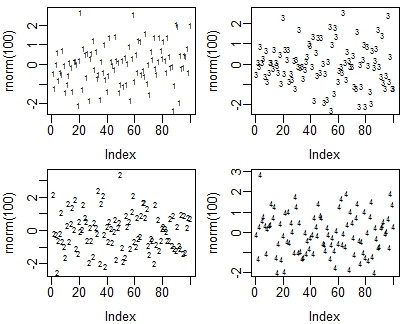```> par(mar=c(2, 2, 0.5, 0.5))
> mat <- matrix(c(1,1,2,3,4,4), nrow=2, byrow=TRUE)
> mat
[,1] [,2] [,3]
[1,]    1    1    2
[2,]    3    4    4
> layout(mat)
> set.seed(1000)
> plot(rnorm(100),pch="1", cex=0.6)
> set.seed(1001)
> plot(rnorm(100),pch="2", cex=0.6)
> set.seed(1002)
> plot(rnorm(100),pch="3", cex=0.6)
> set.seed(1003)
> plot(rnorm(100),pch="4", cex=0.6)```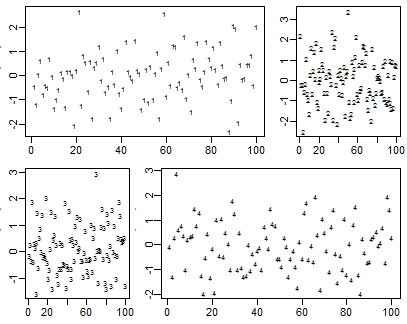```> par(mar=c(2, 2, 0.5, 0.5))
> mat <- matrix(c(1,2,3,4,4,4), nrow=2, byrow=TRUE)
> mat
[,1] [,2] [,3]
[1,]    1    2    3
[2,]    4    4    4
> layout(mat)
> set.seed(1000)
> plot(rnorm(100),pch="1", cex=0.6)
> set.seed(1001)
> plot(rnorm(100),pch="2", cex=0.6)
> set.seed(1002)
> plot(rnorm(100),pch="3", cex=0.6)
> set.seed(1003)
> plot(rnorm(100),pch="4", cex=0.6)```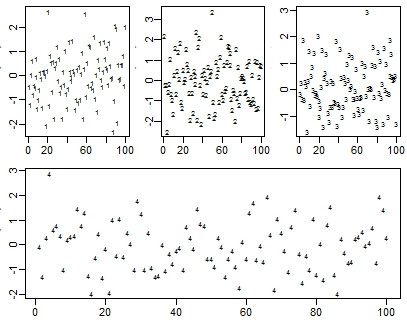• 本文由 发表
• 转载请务必保留本文链接：https://www.plob.org/article/7542.html